## Momentum

• Mass just resting on a surface has inertia.
• Momentum is the inertia of movement.
• Momentum has to do with the mass of the object and its speed.
• The symbol for momentum is "p"

p = mass x velocity
p = mv

• (p gets its name from impetus which comes from the latin "petere" which means "to go towards or rush upon".)
• Units: kg•m/s (no unit that is shortened to like N)
• Momentum is a vector and is in the same direction as the velocity.
• With momentum, establishing a direction as positive or negative is important.

### Sample problem

What is the momentum of a 2kg ball that is thrown at 20m/s?

p = mv

= (2kg)(20m/s)
= 40kg•m/s

Boy! That was easy.
Momentum is quick and easy.

Here endeth the lesson!

## Impulse

Impulse is a measure of how long a force is applied to a mass or more simply put: the change in momentum. Its symbol is J.

For example:

If you have a fixed amount of force, say: 100N. How can you get it to move something faster?

You can get more speed by pulling with the 100N for a longer and longer time.
Acceleration is caused by the net force (Fn) acting on a mass.

a = F/m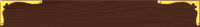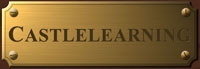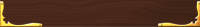Conservation of Momentum
Elastic Collisions
Inelastic Collisions## Worksheets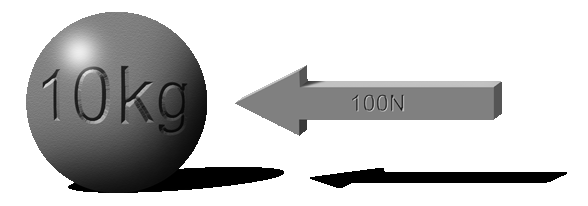This ball will accelerate at 10m/s2. After 1 second it is traveling at 10m/s. After 2 seconds it is traveling at 20m/s. After 3 seconds 30m/s etc. The longer you apply the force, the faster it goes.

a = Fnet/m

also

a = Δv/Δt

Therefore since both acceleration equations are equal to each other:
Fnet/m = Δv/Δt

Which gets rearranged into:

m•Δv = FNet•Δt

As time goes up, the velocity also goes up. (You give the impulse for a longer time, the velocity will get faster.)
The longer you are in contact, the force needed goes down in order to keep that side of the equation worth the same.
As t goes down, F goes up in order to produce the same acceleration.

This means that if you need to go from 55mph down to zero (a car slowing down to a stop) your change in velocity will be the same no matter how you do it. But!:

If you slow down gradually over a long time (gentle braking), you will experience a very small force.
If you slam on the brakes, you stop in a smaller time and you will feel more force against the seatbelt.
If you stop extremely quickly, like hitting a wall*, you will experience a much higher force. The force may exceed the tolerance of your body and things break.

*Professional driver on a closed course. Do not attempt.

Impulse is the change in momentum and the symbol is J.

J = Δp

If you follow through with the equalities:

J = Δp = mΔv = F•t

Keep in mind that if things are equals to each other they can be substituted to take each other's place. This makes it easy to use these equations for many situations depending on what variables you have.

The units for impulse are N•s

p = m•v which gives you kg•m/s which you may have noticed is the same exact units as momentum.

Why is one kg•m/s instead of N•s?
Momentum is describing an object’s inertia of movement. And movement is best described with velocity (m/s)
Impulse is describing how the force acting on the object. Force is best described using Newtons (N).

So, it just depends on how you want to describe the action.

 It's nice to have them all in one spot! a = Δv/t a = F/m p = m•v (units•kg Δm/s) J = Δ p = m Δv = F•t (units: N•s) Δp = pf - pi Δv = vf – vi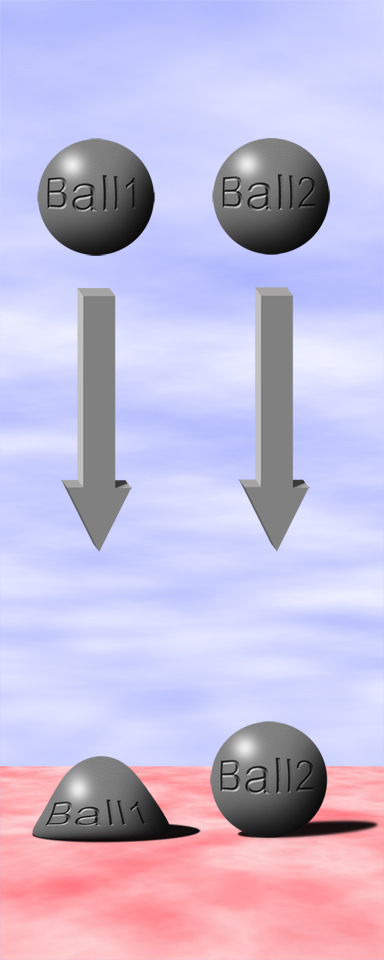With two balls falling: vi is the same for both vf is the same for both soft1 vs hard2 p1 = p2 Δp1 = Δp2 J1 = J2 F1•t1 = F2•t2 Everything about these two dropped balls are the same except how quickly the collision happens. As the time of collision increases (slower, softer collision) the Force decreases. As the time of collision decreases (hard, rigid collision) the Force increases. You can say that a soft collision spreads the impact out over alonger time while a hard collision focuses all the impact at one moment. "It's not the fall that will kill you. It's the sudden stop at the end." -Douglas Adams author of Hitchhiker's Guide to the Universe

### Some examples of collisions:

A baseball hitting a wall filmed by a high speed camera.

In this video you can get some calculations of distance, time and speed along with mass and size of a baseball to make accurate calculations of velocity, momentum and impulse.

A golf ball fired at 150mph (67m/s) filmed at 70000 frames/sec

I believe it's real but you be the judge.

The crumple zone in of a car absorbs the energy of impact by spreading out the time at which the collision takes place.

Here's a car that is ALL crumple zone.

### Conservation of Motion

Whatever momentum you had before an event will be the same as after the event.

pbefore = pafter

If you have zero momentum before say, an explosion, then you will have zero after.
Before an explosion, of course (usually) there is no movement so there is no momentum.
After the explosion, of course (usually) there will be movement but the movement will be in opposite directions and the values will cancel out.

When dealing with momentum, it is really important to keep track of positives and negatives.

### Example of an explosion problem:

A gun fires:
mgun = 2.5kg
mbullet = .025kg
Muzzle velocity = 250m/s
Time to fire = .001sec
What is the velocity of the gun?
What is the force? (same for gun and bullet)

What is the velocity of the gun?

pbefore = pafter
Before(pgun + pbullet) = After(pgun + pbullet)
Before the shot nothing was moving. Velocity for the gun and bullet was zero. Therefore, no momentum for either:

Before(0kg•m/s) = After(pgun + pbullet)

Since the momentums (momenta) after the shot or the gun and bullet add up to equal zero, the two momenta must be equal but opposite of each other.

0 = pgun + pbullet

0 - pgun = pgun + pbullet - pgun (subtract pgun from both sides of the equation)

-(mgun•vgun) = (mbullet•vbullet)

to solve for vgun:

-vgun = mbullet•vbullet/mgun

-vgun = (.025kg)(250m/s) / 2.5kg

-vgun = -2.5m/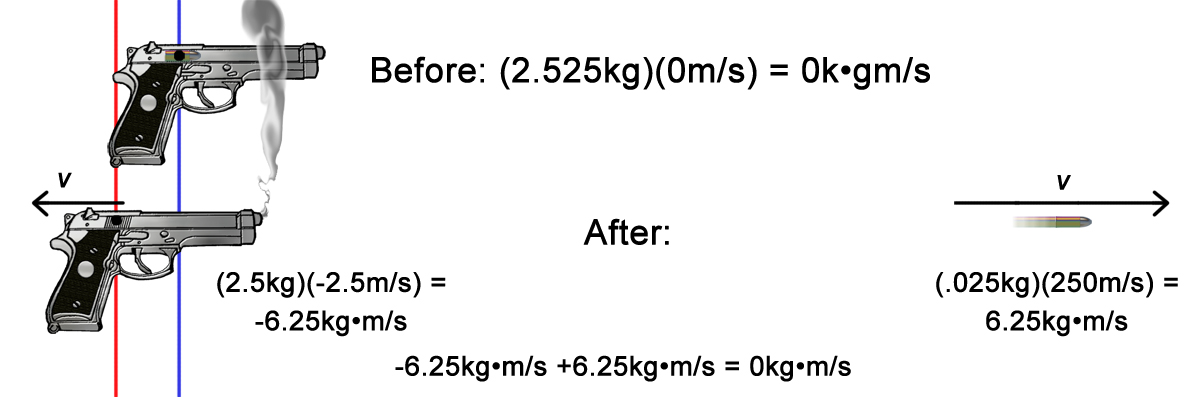What is the force? (same for gun and bullet)

F = ma and a = Δv/Δt

Therefore: F = m•Δv/Δt
F = (.025kg) (250m/s) / (.001s)
F = 6250N (applied for 1/1,000sec)

Some recoils:

Note: The first half of the video shows people who were either not expecting the recoil or were in a very bad stance when firing. They were not thrown off their feet.

In the second half of the video the men are firing a hugely powerful gun. Although it hit them with quite a punch, it actually doesn't knock anyone through the air (but some did trip or stumble). But it's still good fun to watch!

## Elastic Collisions

With an elastic collision, as with all collisions, momentum is conserved. The amount of momentum before the collision must equal the amount of momentum after the collision.

Add up the momenta of both objects including Positive and Negative velocities.
After the collision, the momentum will be the same, and along with the velocity of one of the balls you can calculate the velocity of the second ball.

### Practice Problem:

Two balls collide as shown. A 10kg ball moving at 5m/s collides with a 5kg ball moving at -4m/s.
What is the final velocity of the 5kg ball after the collision?

(In this exercise movement to the right is established as positive and to the left is negative. You can do it any way you wish as long as you are consistent for the entirety of the problem.)

Before:
The blue ball has a momentum of (10kg)(5m/s) or 50kg•m/s.
The red ball has a momentum of (5kg)(-4m/s) or -20kg•m/s.

The total momentum before the collision is 50kg•m/s + (-20kg•m/s) or 30kg•m/s.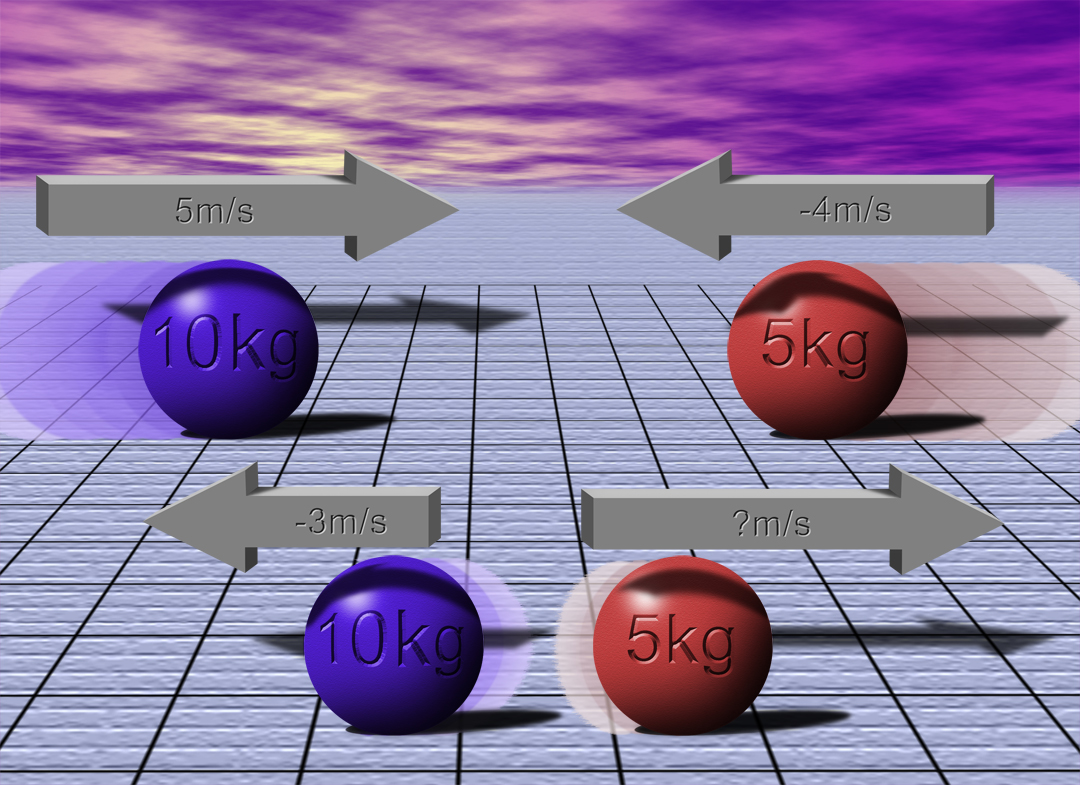After the collision they must have the same momentum: 30kg•m/s.

After:
The blue ball has bounced off the red and is now heading in the opposite direction.
The momentum of the blue ball is now (10kg)(-3m/s) or -30kg•m/s.
The red ball has an unknown velocity so it’s momentum will be (5kg)(?m/s).

To solve:
pbefore = pafter

30 kg•m/s = -30 kg•m/s + (5kg)(?m/s)
+30 kg•m/s +30 kg•m/s

60 kg•m/s = (5kg)(?m/s)

Divide both sides by 5kg:
60kg•m/s/5kg = ?m/s

12m/s = vfinal

## Inelastic (Sticky) Collisions

With a “sticky” collision (inelastic) the same rule applies: momentum before must equal the momentum after. However, in this case, neither object will reverse direction. After the collision, they will act as one mass and “share” the original momentum.

### Practice Problem:

A 15kg block of ice is sitting at rest on a frozen lake. A 3kg snowball is thrown at the block at a velocity of 35m/s. When the snowball hits the block, the two of them stick together. Assuming that ice on ice is frictionless, what is the final velocity of the block-snowball combination?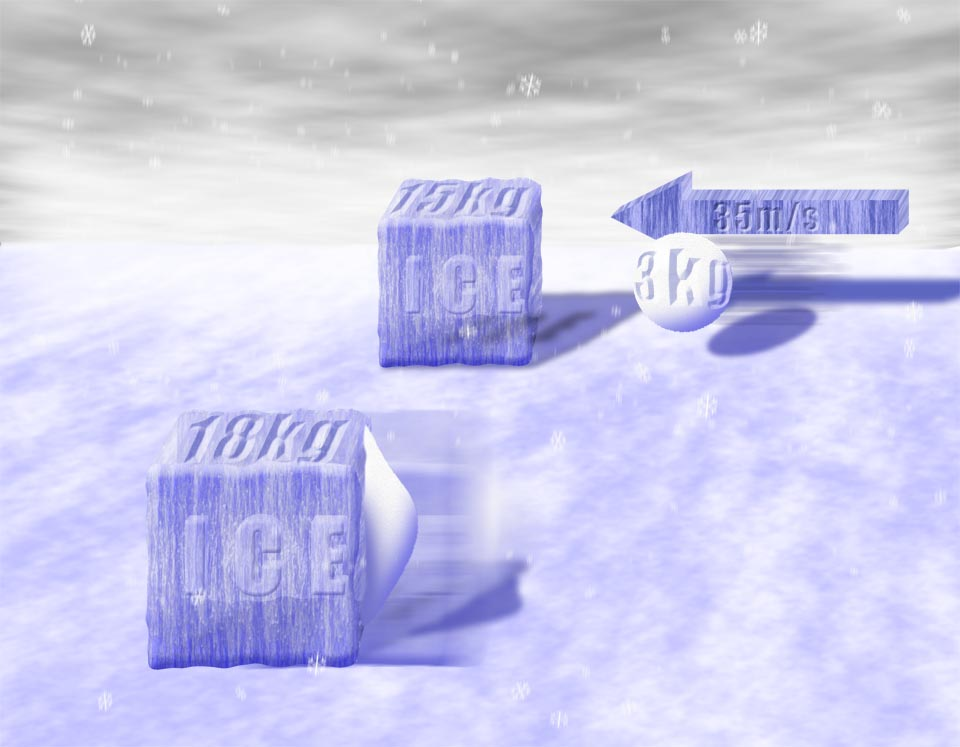Before the collision the momentum of the system is:
p = (15kg)(0m/s) + (3kg)(35m/s)
p = 105kg •m/s

After the collision the two objects together must have 105kg •m/s of momentum.
105kg •m/s = (18kg)(?m/s)
105kg •m/s / 18kg = v
5.8m/s = vMedina On-Line
Since 1994
Email: mrsciguy@optonline.net